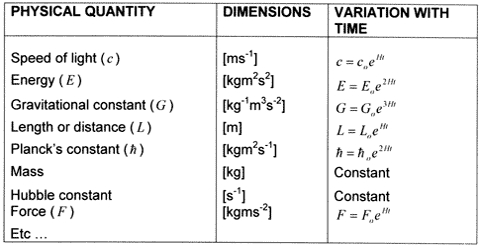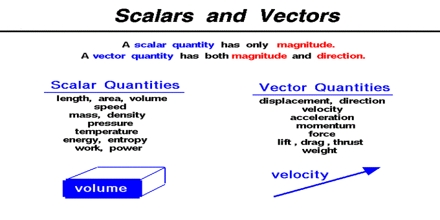# Physical Quantity

In order to know definitely different aspects of science, one or the other form of measurement is needed. Physical characteristics of matter that can be measured are called quantities. As for example, each of the terms such as length, mass, time, volume, velocity, work etc is a quantity. Any quantity in physics is called physical quantity. A physical quantity is a property of an object that can be measured with a measuring instrument.For example length, width, time, weight, etc.

Some physical quantities can be expressed only by magnitude. On the other hand there are many physical quantities which can completely be expressed by both magnitude and direction. Hence, according to properties or characteristics we can classify physical quantifies into two categories: (a) Scalar quantity and (b) Vector quantity.Scalar quantity: A physical quantity which has oak magnitude and no direction is called scalar quantity or scalar. For example: length, mass, time, population, temperature, heat, electric potential, speed etc are scalar quantities.

Vector quantity: A physical quantity which has both magnitude and direction is called vector quantity or vector. For example: displacement, velocity, acceleration, deceleration or retardation, force, weight etc are vector quantities.